Chapter 8 Electromagnetic Induction, AC Circuits, and Electrical Technologies

# 8.3 Motional Emf

### Summary

• Calculate emf, force, magnetic field, and work due to the motion of an object in a magnetic field.

As we have seen, any change in magnetic flux induces an emf opposing that change—a process known as induction. Motion is one of the major causes of induction. For example, a magnet moved toward a coil induces an emf, and a coil moved toward a magnet produces a similar emf. In this section, we concentrate on motion in a magnetic field that is stationary relative to the Earth, producing what is loosely called motional emf.

One situation where motional emf occurs is known as the Hall effect and has already been examined. Charges moving in a magnetic field experience the magnetic force F = qvB sin θ, which moves opposite charges in opposite directions and produces an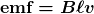. We saw that the Hall effect has applications, including measurements of B and v. We will now see that the Hall effect is one aspect of the broader phenomenon of induction, and we will find that motional emf can be used as a power source.

Consider the situation shown in Figure 1. A rod is moved at a speed v along a pair of conducting rails separated by a distance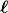in a uniform magnetic field B. The rails are stationary relative to B and are connected to a stationary resistor R. The resistor could be anything from a light bulb to a voltmeter. Consider the area enclosed by the moving rod, rails, and resistor. B is perpendicular to this area, and the area is increasing as the rod moves. Thus the magnetic flux enclosed by the rails, rod, and resistor is increasing. When flux changes, an emf is induced according to Faraday’s law of induction.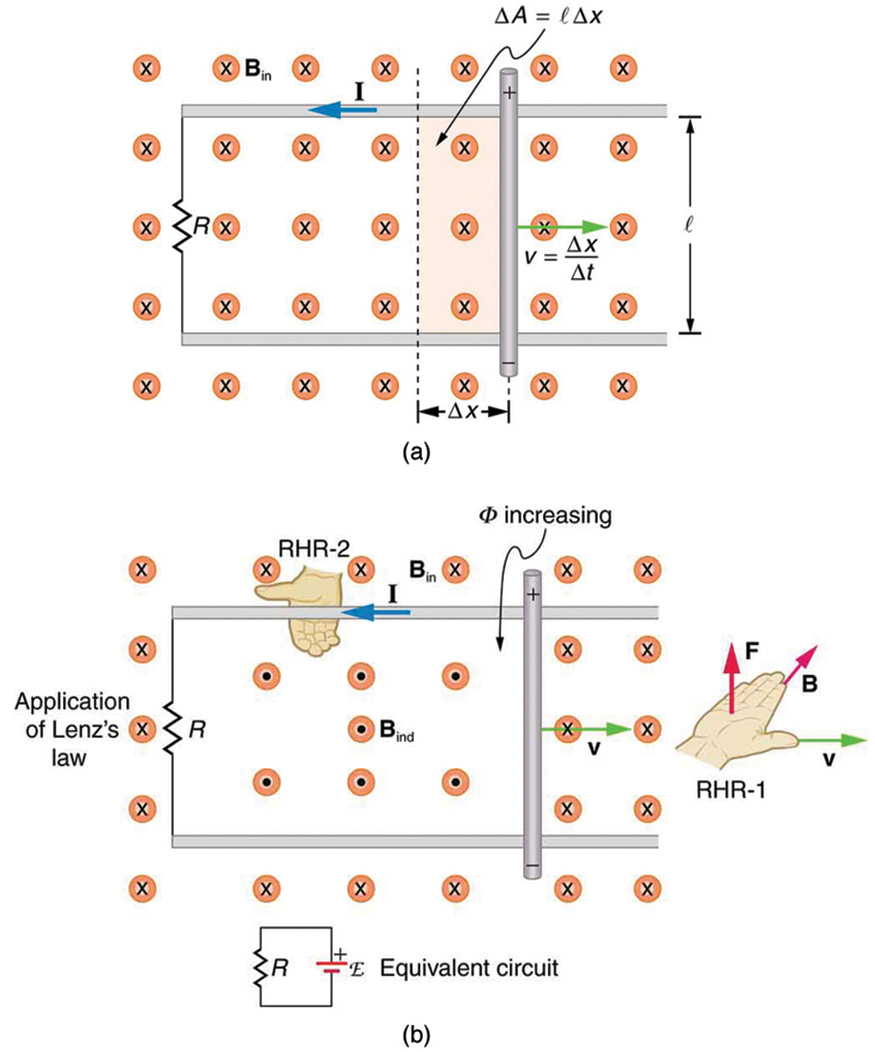Figure 1. (a) A motional emf = Bℓv is induced between the rails when this rod moves to the right in the uniform magnetic field. The magnetic field B is into the page, perpendicular to the moving rod and rails and, hence, to the area enclosed by them. (b) Lenz’s law gives the directions of the induced field and current, and the polarity of the induced emf. Since the flux is increasing, the induced field is in the opposite direction, or out of the page. RHR-2 gives the current direction shown, and the polarity of the rod will drive such a current. RHR-1 also indicates the same polarity for the rod. (Note that the script E symbol used in the equivalent circuit at the bottom of part (b) represents emf.)

To find the magnitude of emf induced along the moving rod, we use Faraday’s law of induction without the sign: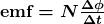.

Here and below, “emf” implies the magnitude of the emf. In this equation, N = 1 and the flux φ= BA cosθ. We have θ=0o and cos θ=1, since B is perpendicular to A. Now ΔφΔBA = B ΔA, since is uniform. Note that the area swept out by the rod is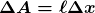. Entering these quantities into the expression for emf yields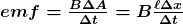Finally, note that v = Δ x / Δt  , the velocity of the rod. Entering this into the last expression shows that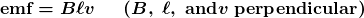is the motional emf. This is the same expression given for the Hall effect previously.

### Making Connections: Unification of Forces

There are many connections between the electric force and the magnetic force. The fact that a moving electric field produces a magnetic field and, conversely, a moving magnetic field produces an electric field is part of why electric and magnetic forces are now considered to be different manifestations of the same force. This classic unification of electric and magnetic forces into what is called the electromagnetic force is the inspiration for contemporary efforts to unify other basic forces.

To find the direction of the induced field, the direction of the current, and the polarity of the induced emf, we apply Lenz’s law as explained in Faraday’s Law of Induction: Lenz’s Law. (See Figure 1(b).) Flux is increasing, since the area enclosed is increasing. Thus the induced field must oppose the existing one and be out of the page. And so the RHR-2 requires that I be counterclockwise, which in turn means the top of the rod is positive as shown.

Motional emf also occurs if the magnetic field moves and the rod (or other object) is stationary relative to the Earth (or some observer). We have seen an example of this in the situation where a moving magnet induces an emf in a stationary coil. It is the relative motion that is important. What is emerging in these observations is a connection between magnetic and electric fields. A moving magnetic field produces an electric field through its induced emf. We already have seen that a moving electric field produces a magnetic field—moving charge implies moving electric field and moving charge produces a magnetic field.

Motional emfs in the Earth’s weak magnetic field are not ordinarily very large, or we would notice voltage along metal rods, such as a screwdriver, during ordinary motions. For example, a simple calculation of the motional emf of a 1 m rod moving at 3.0 m/s perpendicular to the Earth’s field gives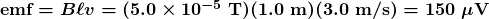. This small value is consistent with experience. There is a spectacular exception, however. In 1992 and 1996, attempts were made with the space shuttle to create large motional emfs. The Tethered Satellite was to be let out on a 20 km length of wire as shown in Figure 2, to create a 5 kV emf by moving at orbital speed through the Earth’s field. This emf could be used to convert some of the shuttle’s kinetic and potential energy into electrical energy if a complete circuit could be made. To complete the circuit, the stationary ionosphere was to supply a return path for the current to flow. (The ionosphere is the rarefied and partially ionized atmosphere at orbital altitudes. It conducts because of the ionization. The ionosphere serves the same function as the stationary rails and connecting resistor in Figure 1, without which there would not be a complete circuit.) Drag on the current in the cable due to the magnetic force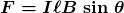does the work that reduces the shuttle’s kinetic and potential energy and allows it to be converted to electrical energy. The tests were both unsuccessful. In the first, the cable hung up and could only be extended a couple of hundred meters; in the second, the cable broke when almost fully extended. Example 1 indicates feasibility in principle.

### Example 1: Calculating the Large Motional Emf of an Object in Orbit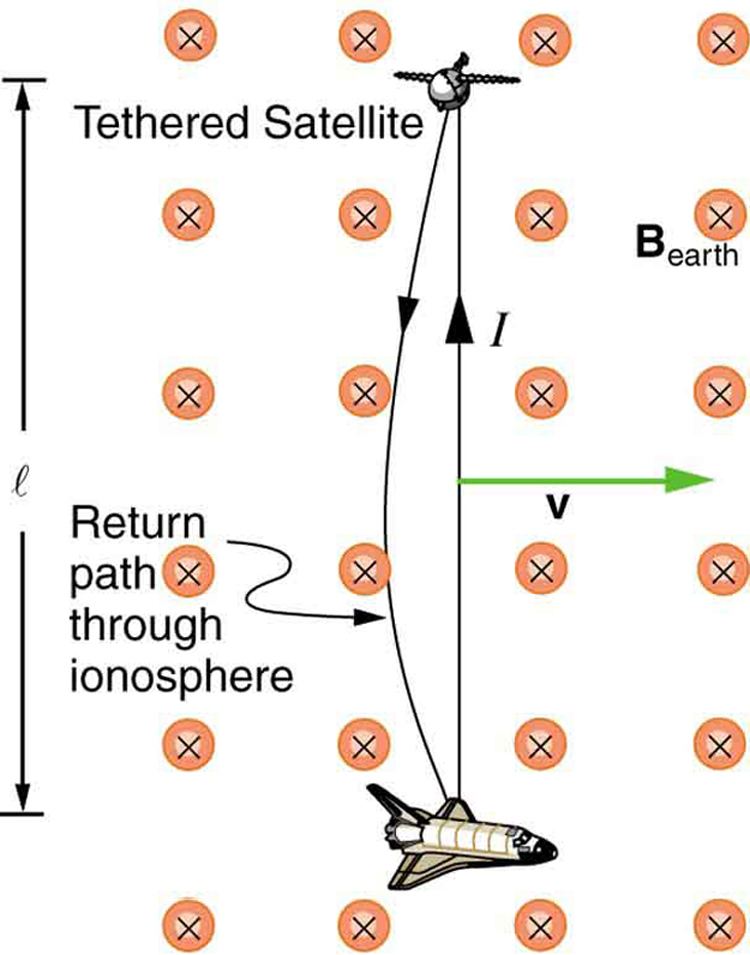Figure 2. Motional emf as electrical power conversion for the space shuttle is the motivation for the Tethered Satellite experiment. A 5 kV emf was predicted to be induced in the 20 km long tether while moving at orbital speed in the Earth’s magnetic field. The circuit is completed by a return path through the stationary ionosphere.

Calculate the motional emf induced along a 20.0 km long conductor moving at an orbital speed of 7.80 km/s perpendicular to the Earth’s 5.00 x 10-5 T magnetic field.

Strategy

This is a straightforward application of the expression for motional emf—.

Solution

Entering the given values intogives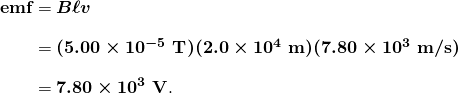Discussion

The value obtained is greater than the 5 kV measured voltage for the shuttle experiment, since the actual orbital motion of the tether is not perpendicular to the Earth’s field. The 7.80 kV value is the maximum emf obtained when θ = 90o   and  sinθ=1.

# Section Summary

• An emf induced by motion relative to a magnetic field is called a motional emf and is given by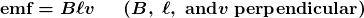whereis the length of the object moving at speed relative to the field.

### Conceptual Questions

1: Why must part of the circuit be moving relative to other parts, to have usable motional emf? Consider, for example, that the rails in Figure 1 are stationary relative to the magnetic field, while the rod moves.

2: A powerful induction cannon can be made by placing a metal cylinder inside a solenoid coil. The cylinder is forcefully expelled when solenoid current is turned on rapidly. Use Faraday’s and Lenz’s laws to explain how this works. Why might the cylinder get live/hot when the cannon is fired?

3: An induction stove heats a pot with a coil carrying an alternating current located beneath the pot (and without a hot surface). Can the stove surface be a conductor? Why won’t a coil carrying a direct current work?

4: Explain how you could thaw out a frozen water pipe by wrapping a coil carrying an alternating current around it. Does it matter whether or not the pipe is a conductor? Explain.

### Problems & Exercises

1: Use Faraday’s law, Lenz’s law, and RHR-1 to show that the magnetic force on the current in the moving rod in Figure 1 is in the opposite direction of its velocity.

2: If a current flows in the Satellite Tether shown in Figure 2, use Faraday’s law, Lenz’s law, and RHR-1 to show that there is a magnetic force on the tether in the direction opposite to its velocity.

3: (a) A jet airplane with a 75.0 m wingspan is flying at 280 m/s. What emf is induced between wing tips if the vertical component of the Earth’s field is  3.00 x10-5 T. ? (b) Is an emf of this magnitude likely to have any consequences? Explain.

4: (a) A nonferrous screwdriver is being used in a 2.00 T magnetic field. What maximum emf can be induced along its 12.0 cm length when it moves at 6.00 m/s? (b) Is it likely that this emf will have any consequences or even be noticed?

5: At what speed must the sliding rod in Figure 1 move to produce an emf of 1.00 V in a 1.50 T field, given the rod’s length is 30.0 cm?

6: The 12.0 cm long rod in Figure 1 moves at 4.00 m/s. What is the strength of the magnetic field if a 95.0 V emf is induced?

7: Prove that when ,, and v are not mutually perpendicular, motional emf is given by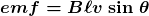. If v is perpendicular to B, then θ is the angle betweenand B. Ifis perpendicular to B, then θ is the angle between v and B.

8: In the August 1992 space shuttle flight, only 250 m of the conducting tether considered in Example 1 could be let out. A 40.0 V motional emf was generated in the Earth’s 5.00 x 10-5 T field, while moving at 7.80 x 103 m/s . What was the angle between the shuttle’s velocity and the Earth’s field, assuming the conductor was perpendicular to the field?

9: Integrated Concepts

Derive an expression for the current in a system like that in Figure 2, under the following conditions. The resistance between the rails is R, the rails and the moving rod are identical in cross section A and have the same resistivity ρ. The distance between the rails is l, and the rod moves at constant speed v perpendicular to the uniform field B. At time zero, the moving rod is next to the resistance R.

10: Integrated Concepts

The Tethered Satellite in Figure 2 has a mass of 525 kg and is at the end of a 20.0 km long, 2.50 mm diameter cable with the tensile strength of steel. (a) How much does the cable stretch if a 100 N force is exerted to pull the satellite in? (Assume the satellite and shuttle are at the same altitude above the Earth.) (b) What is the effective force constant of the cable? (c) How much energy is stored in it when stretched by the 100 N force?

11: Integrated Concepts

The Tethered Satellite discussed in this module is producing 5.00 kV, and a current of 10.0 A flows. (a) What magnetic drag force does this produce if the system is moving at 7.80 km/s? (b) How much kinetic energy is removed from the system in 1.00 h, neglecting any change in altitude or velocity during that time? (c) What is the change in velocity if the mass of the system is 100,000 kg? (d) Discuss the long term consequences (say, a week-long mission) on the space shuttle’s orbit, noting what effect a decrease in velocity has and assessing the magnitude of the effect.

### Solutions

Problems & Exercises

3: (a) 0.630 V (b) No, this is a very small emf.

5: 2.22 m/s

11: (a) 10.0 N (b)  2.81 x 108 J  (c) 0.36 m/s  (d) For a week-long mission (168 hours), the change in velocity will be 60 m/s, or approximately 1%. In general, a decrease in velocity would cause the orbit to start spiralling inward because the velocity would no longer be sufficient to keep the circular orbit. The long-term consequences are that the shuttle would require a little more fuel to maintain the desired speed, otherwise the orbit would spiral slightly inward.